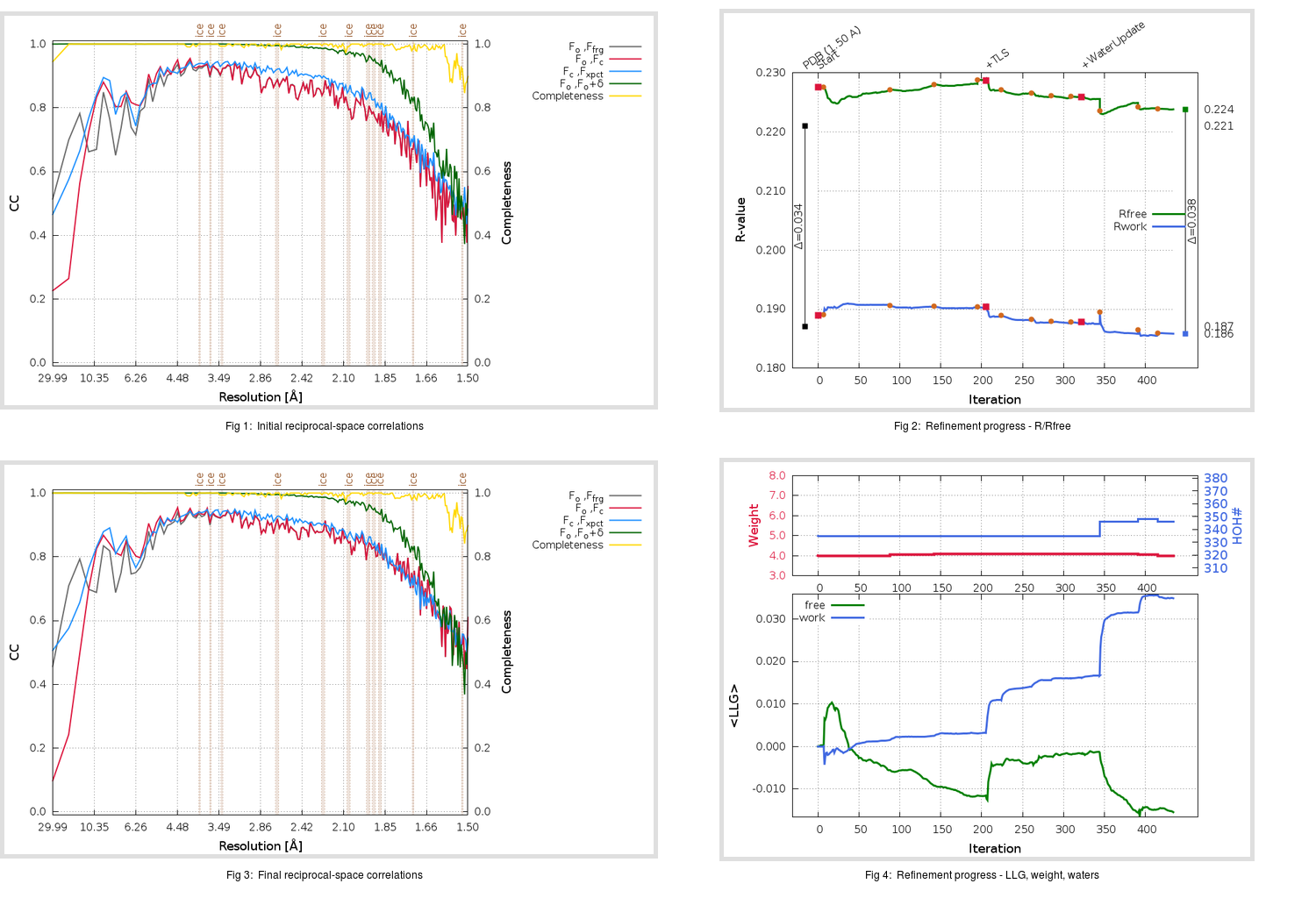Content:

```    Diffraction limits & principal axes of ellipsoid fitted to diffraction cut-off surface:
1.774         0.9677   0.0000  -0.2522       0.982 a* - 0.187 c*
1.490         0.0000   1.0000   0.0000       b*
1.471         0.2522   0.0000   0.9677       0.584 a* + 0.812 c*
```

## Deposited

` `
 Date deposited Date data collection Resolution R, Rfree 20200315 20200305 1.50 0.1850 0.2210

Molprobity (CCP4 7.0 version) summary:

```Ramachandran outliers =   0.33 %
favored =  97.68 %
Rotamer outliers      =   0.38 %
C-beta deviations     =     0
Clashscore            =   3.60
RMS(bonds)            =   0.0130
RMS(angles)           =   1.80
MolProbity score      =   1.22
Resolution            =   1.50
R-work                =   0.1850
R-free                =   0.2210
```

```Number of waters      =   335

<B> (all atoms) =   21.42 ( sd =    7.97 ) for       2724 non-hydrogen atoms
<B>   (protein) =   19.92 ( sd =    6.73 ) for       2370 non-hydrogen atoms
<B>     (water) =   31.79 ( sd =    8.61 ) for        335 non-hydrogen atoms
<B>    (others) =   28.25 ( sd =    5.07 ) for         19 non-hydrogen atoms

B min/max       (all non-hydrogen atoms) =   10.63 /   59.36
B min/max   (protein non-hydrogen atoms) =   10.63 /   59.36
B min/max     (water non-hydrogen atoms) =   11.68 /   53.03
B min/max     (other non-hydrogen atoms) =   23.86 /   40.12
```

## BUSTER (re-)refinement

` `

Molprobity (CCP4 7.0 version) summary:

```Ramachandran outliers =   0.33 %
favored =  98.34 %
Rotamer outliers      =   1.14 %
C-beta deviations     =     0
Clashscore            =   2.54
RMS(bonds)            =   0.0112
RMS(angles)           =   1.57
MolProbity score      =   1.08
Resolution            =   1.50
R-work                =   0.1859
R-free                =   0.2238
```

```Number of waters      =   346

<B> (all atoms) =   23.51 ( sd =    8.22 ) for       2735 non-hydrogen atoms
<B>   (protein) =   21.70 ( sd =    6.20 ) for       2370 non-hydrogen atoms
<B>     (water) =   35.55 ( sd =    9.96 ) for        346 non-hydrogen atoms
<B>    (others) =   29.60 ( sd =    5.35 ) for         19 non-hydrogen atoms

B min/max       (all non-hydrogen atoms) =    7.46 /   68.91
B min/max   (protein non-hydrogen atoms) =   13.30 /   50.66
B min/max     (water non-hydrogen atoms) =    7.46 /   68.91
B min/max     (other non-hydrogen atoms) =   24.45 /   39.28
```

Refinement progression:Results:

` `
 File Remark 5RF3_aB_refine.01_03_refine.pdb.gz exact refinement commands are in header 5RF3_aB_refine.01_03_refine.mtz.gz including original deposited data and several re-refinement map coefficients 5RF3_aB_refine.01_03_BUSTER_model.cif.gz including any non-standard compound restraints 5RF3_aB_refine.01_03_BUSTER_refln.cif.gz